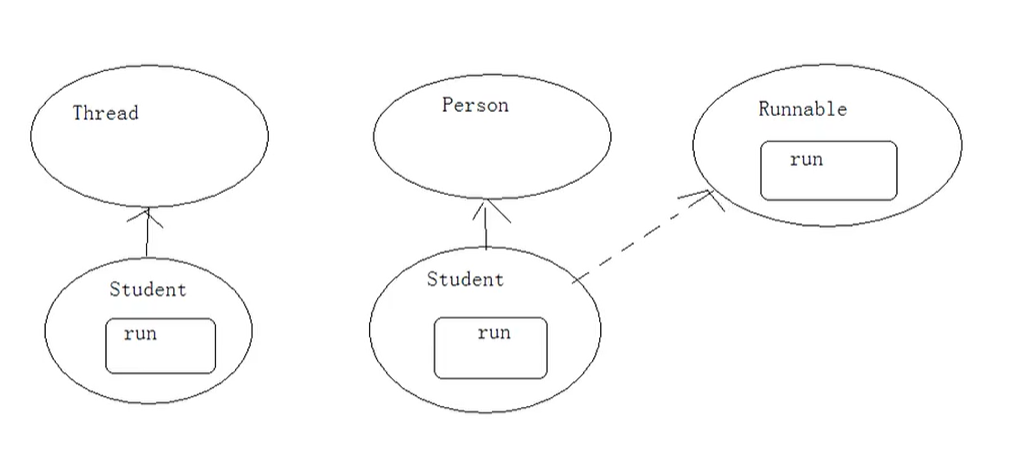毕25-第六天（day11）-多线程

17 篇文章 0 订阅
• 多线程创建及运行

JavaVM启动的时候会有一个进程java.exe，该进程中至少有一个线程负责Java程序的执行，而且这个线程运行的代码存在于main方法中，该线程称之为主线程。

public void run() {
for(int x=0; x<60; x++)
System.out.println("demo run.."+x);
}
}

public class Main{
public static void main(String[] args) {
Demo d = new Demo();
d.start();
for(int x=0; x<60; x++)
System.out.println("main run.."+x);
}
}

• 获取线程对象及名称

Test(String name){
}
public void run() {
for(int x=0; x<60; x++) {
System.out.println(this.getName()+"run.."+x);
}
}
}

public class Main{
public static void main(String[] args) {
Test t1 = new Test("one");
Test t2 = new Test("two");
t1.start();
t2.start();
for(int x=0; x<60; x++) {
System.out.println("main run.."+x);
}
}
}

getName()：获取线程名称。

• 卖票小程序

private static int tick = 100;
public void run() {
while(true) {
if(tick>0) {
}
}
}
}

public class Main{
public static void main(String[] args) {
Ticket t1 = new Ticket();
Ticket t2 = new Ticket();
Ticket t3 = new Ticket();
Ticket t4 = new Ticket();
t1.start();
t2.start();
t3.start();
t4.start();
}
}

class Ticket implements Runnable{
private int tick = 100;
public void run() {
while(true) {
if(tick>0) {
}
}
}
}

public class Main{
public static void main(String[] args) {
Ticket t = new Ticket();
t1.start();
t2.start();
t3.start();
t4.start();
}
}

1、定义类实现Runnable接口；

2、覆盖Runnable接口中的run方法，将线程要运行的代码存放在该run方法中；• 多线程的安全问题

class Ticket implements Runnable{
private int tick = 100;
public void run() {
while(true) {
if(tick>0) {
}catch(Exception e){}
}
}
}
}

public class Main{
public static void main(String[] args) {
Ticket t = new Ticket();
t1.start();
t2.start();
t3.start();
t4.start();
}
}

Java对于多线程的安全问题提供了专业的解决方式，就是同步代码块：

synchronized(对象){
//需要被同步的代码；
}

class Ticket implements Runnable{
private int tick = 100;
Object obj = new Object();
public void run() {
while(true) {
synchronized(obj) {    //同步代码块
if(tick>0) {
}catch(Exception e){}
}
}
}
}
}

public class Main{
public static void main(String[] args) {
Ticket t = new Ticket();
t1.start();
t2.start();
t3.start();
t4.start();
}
}

1、必须要有两个或两个以上的线程；

2、必须是多个线程使用同一个锁。

• 同步函数

/*

*/
class Bank{
private int sum;
sum = sum+n;
System.out.println("sum="+sum);
}
}

class Save implements Runnable{
private Bank b = new Bank();
public void run() {
for(int x=0; x<3; x++) {
}
}
}

public class Main{
public static void main(String[] args) {
Save s = new Save();
t1.start();
t2.start();
}
}

sum=100
sum=200
sum=300
sum=400
sum=500
sum=600

1、明确那些代码是多线程代码；

2、明确共享数据；

3、明确多线程运行代码中哪些语句是操作共享数据的。

class Bank{
private int sum;
sum = sum+n;
System.out.println("sum="+sum);
}
}

sum=200
sum=200
sum=400
sum=400
sum=600
sum=600

class Bank{
private int sum;
Object obj = new Object();
synchronized(obj){
sum = sum+n;
System.out.println("sum="+sum);
}
}
}

class Bank{
private int sum;
public synchronized void add(int n) {
sum = sum+n;
System.out.println("sum="+sum);
}
}
• 同步函数的锁是this

卖票例子的同步函数版本：

class Ticket implements Runnable{
private int tick = 100;
public void run() {		//不可以给run方法上锁，这是因为一旦给润、上锁了，这时第一个线程进来了，后面的线程都进不来了
while(true) {
show();
}
}
public synchronized void show() {
if(tick>0) {
}
}
}

public class Main{
public static void main(String[] args) {
Ticket t = new Ticket();
t1.start();
t2.start();
t3.start();
t4.start();
}
}

class Ticket implements Runnable{
private int tick = 100;
Object obj = new Object();
boolean flag = true;
public void run() {
if(flag) {
while(true) {
synchronized(obj) {
if(tick>0) {
}
}
}
}else
while(true)
show();
}
public synchronized void show() {
if(tick>0) {
}
}
}

public class Main{
public static void main(String[] args) {
Ticket t = new Ticket();
t1.start();
t.flag = false;
t2.start();
}
}

• 静态同步函数的锁是Class对象

class Ticket implements Runnable{
private static int tick = 100;
boolean flag = true;
public void run() {
if(flag) {
while(true) {
synchronized(Ticket.class) {
if(tick>0) {
}
}
}
}else
while(true)
show();
}
public static synchronized void show() {
if(tick>0) {
}
}
}

public class Main{
public static void main(String[] args) {
Ticket t = new Ticket();
t1.start();
t.flag = false;
t2.start();
}
}

08-0418
08-111612-2623
08-0414
08-1129
05-2838
03-3084
08-0519
11-3022
03-1339
08-172
06-0510
05-2461
01-1554
04-0830
05-2516
06-0323
07-1314
01-21sleepingposture

¥2 ¥4 ¥6 ¥10 ¥20余额支付 (余额：-- )扫码支付获取中扫码支付点击重新获取扫码支付1.余额是钱包充值的虚拟货币，按照1:1的比例进行支付金额的抵扣。
2.余额无法直接购买下载，可以购买VIP、C币套餐、付费专栏及课程。余额充值